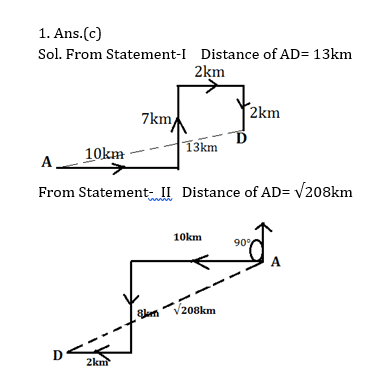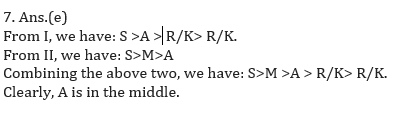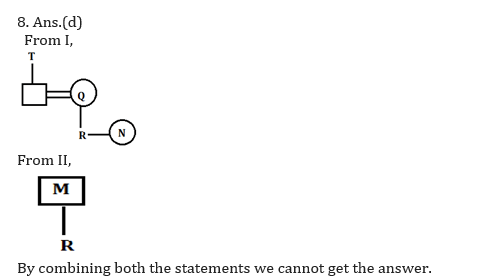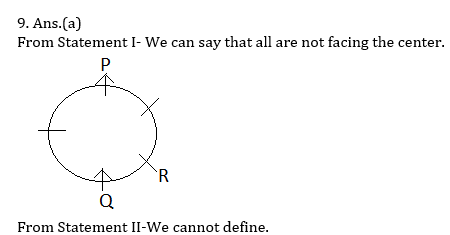# Data Sufficiency for IBPS, SBI, RBI, RRBs: Quiz 2

## Data Sufficiency for IBPS, SBI, RBI, RRBs: Quiz 2

Data Sufficiency plays a significant role in Reasoning Ability Section of banking exams such as IBPS, SBI and RBI PO and Clerk. You will get at least 5 questions from Data Sufficiency in one of IBPS, SBI and RBI PO & clerk prelims and Mains exam. So, aspirants should focus on Data Sufficiency questions in detail. Here, we are providing you with the Data Sufficiency questions quiz with the detailed solution so that you can easily prepare for Data Sufficiency questions. We are providing here all-important latest pattern-based questions and Previous Year Questions of Data Sufficiency of various Government Exam like IBPS, SBI, and RBI PO and Clerk exam. This Data Sufficiency quiz we are providing is free. Attempt this Data Sufficiency quiz to practice important questions with answers and solutions. And score better in IBPS, SBI and RBI PO and Clerk exam.

Data Sufficiency Quiz to improve your Reasoning Ability for SBI Po & SBI clerk exam IBPS PO Reasoning, IBPS Clerk Reasoning, IBPS RRB Reasoning, LIC AAO, LIC Assistant and another competitive exam.

Directions (1-5): Each of the questions given below consists of a question and two statements numbered I and II. You have to decide whether the data provided in the statements are sufficient to answer the question.

Q1. What is the shortest distance between point A and point D?

I. A person faces North at point A, he walks 10km to his right from point A, after that he takes left turn and walks 7000m , again he walks 2km in his right. Finally he takes right turn walks 2km and reached at point D.

II. A person faces North at point A, and he turns 90° in anticlockwise direction from point A and walked 10km. After that he takes left turn and walks 8km. Finally he takes right turn walks 2km and reached at point D.

(a) If statement I alone is sufficient to answer the question, but statement II by itself is not sufficient to answer the question.

(b) If statement II by itself is sufficient to answer the question, but statement I alone is not sufficient to answer the question.

(c) If statement either I or II is sufficient to answer the question.

(d) If both the statements I and II taken together are not sufficient to answer the question.

(e) If both the statements I and II taken together are sufficient to answer the question.Q2. In a six-storey building (consisting of floors number 1 to wherein the topmost floor is number 6 and the ground floor is number 1) each of the six friends, namely Z, Y, X, W, V and U, lives on a different floor (not necessarily in the same order). Who amongst them lives on the lowermost floor?

I. Z lives on floor number five. Only two persons live between Z and Y.V lives immediately above W.

II. W lives on floor number three. Only two persons live between W and X. Y lives immediately above U. Y lives on an even-numbered floor.

(a) If statement I alone is sufficient to answer the question, but statement II by itself is not sufficient to answer the question.

(b) If statement II by itself is sufficient to answer the question, but statement I alone is not sufficient to answer the question.

(c) If statement either I or II is sufficient to answer the question.

(d) If both the statements I and II taken together are not sufficient to answer the question.

(e) If both the statements I and II taken together are sufficient to answer the question.Q3. Who amongst the following is the greatest?

I.B is less than only 2 persons. A is greater than B. C is greater than E.

II. Both C and E are less than A. C is less than B.

(a) If statement I alone is sufficient to answer the question, but statement II by itself is not sufficient to answer the question.

(b) If statement II by itself is sufficient to answer the question, but statement I alone is not sufficient to answer the question.

(c) If statement either I or II is sufficient to answer the question.

(d) If both the statements I and II taken together are not sufficient to answer the question.

(e) If both the statements I and II taken together are sufficient to answer the question.

1. Ans.(d) We can’t find that who is greatest even from both the statements taking together.

Q4. What is the distance between a School and a park?

I. Starting from the school, Sam walks 10 km in a straight line in south direction. He then turns to his left and covers a distance of 5 km. He again turns to his left and covers a distance of 10 km. He finally turns to his right and covers a certain distance and reaches the park.

II. The park is in North- West direction with respect to school.

(a) If statement I alone is sufficient to answer the question, but statement II by itself is not sufficient to answer the question.

(b) If statement II by itself is sufficient to answer the question, but statement I alone is not sufficient to answer the question.

(c) If statement either I or II is sufficient to answer the question.

(d) If both the statements I and II taken together are not sufficient to answer the question.

(e) If both the statements I and II taken together are sufficient to answer the question.

4.Ans.(d)  Total distance between school and park can’t be determined. From I & II we can’t determine the distance

Q5. What is the code for ‘sky’ in a code language?

I. In that language `jo ko ni sa’ means ‘Sky is the limit’ and ‘jo to ni ja’ means ‘Sky color is blue’.

II. In the same language ‘jo ko ta na’ means ‘Sky has no limit’.

(a) If statement I alone is sufficient to answer the question, but statement II by itself is not sufficient to answer the question.

(b) If statement II by itself is sufficient to answer the question, but statement I alone is not sufficient to answer the question.

(c) If statement either I or II is sufficient to answer the question.

(d) If both the statements I and II taken together are not sufficient to answer the question.

(e) If both the statements I and II taken together are sufficient to answer the question.

1. Ans.(e) From Statement- I, we can find that ‘Sky is’ coded as ‘jo ko’.

From Statement – II, we can find that ‘Sky limit’ is coded as ‘jo ni’.

When we combine both statements we can find that ‘Sky’ is coded as ‘jo’.

Directions (6-10): In each of the questions below consists of a question and two statements numbered I and II given below it. You have to decide whether the data provided in the statements are sufficient to answer the question. Read both the statements and Give answer-

Q6. How is ‘Name’ coded in the code language?
Statements:
I. ‘Jolly Kotak Norway’ means ‘@ # &’ and ‘Name Kotak Just’ means ‘\$ * #’.
II. ‘Name Youth Sometime’ means ‘* %  !’ and ‘Just Norway Sometime’ means ‘\$ @ !’.

(a) If the data in statement I alone are sufficient to answer the question, while the data in statement II alone are not sufficient to answer the question.

(b) If the data in statement II alone are sufficient to answer the question, while the data in statement I alone are not sufficient to answer the question.
(c) If the data either in statement I alone or in statement II alone are sufficient to answer the question
(d) If the data given in both statements I and II together are not sufficient to answer the question.
(e) If the data in both statements I and II together are necessary to answer the question.

1. Ans.(e), To get the code of Name we have to use both the given statements together. From Statement I we can find that Name either coded as \$ or * but from Statement I and II we can clearly find the code of Name i.e. *.

Q7. Who among R, S, K, A, and M is exactly in the middle when they are arranged in ascending order of their heights?
Statements:
I. A is taller than both R and K but shorter than S.
II. M is shorter than S but taller than A.

(a) If the data in statement I alone are sufficient to answer the question, while the data in statement II alone are not sufficient to answer the question.

(b) If the data in statement II alone are sufficient to answer the question, while the data in statement I alone are not sufficient to answer the question.
(c) If the data either in statement I alone or in statement II alone are sufficient to answer the question
(d) If the data given in both statements I and II together are not sufficient to answer the question.
(e) If the data in both statements I and II together are necessary to answer the question.Q8. How is R related to N?
Statements:
I. Q is daughter in law of T. Q has only two child R and N, who is sister of R.
II. M is father of R, who has no brother.

(a) If the data in statement I alone are sufficient to answer the question, while the data in statement II alone are not sufficient to answer the question.

(b) If the data in statement II alone are sufficient to answer the question, while the data in statement I alone are not sufficient to answer the question.
(c) If the data either in statement I alone or in statement II alone are sufficient to answer the question
(d) If the data given in both statements I and II together are not sufficient to answer the question.
(e) If the data in both statements I and II together are necessary to answer the question.Q9. Are all the five friends, viz, P, Q, R, S and T, who are seated around a circular table, facing the centre?

I. P sits second to the left of Q. Q faces the centre. R sits second to the right of P.

II. S sits third to the left of T. T faces the centre. Q sits on the immediate left of S. T is an immediate neighbour of Q.

(a) If the data in statement I alone are sufficient to answer the question, while the data in statement II alone are not sufficient to answer the question.

(b) If the data in statement II alone are sufficient to answer the question, while the data in statement I alone are not sufficient to answer the question.
(c) If the data either in statement I alone or in statement II alone are sufficient to answer the question
(d) If the data given in both statements I and II together are not sufficient to answer the question.
(e) If the data in both statements I and II together are necessary to answer the question.Q10. Find the number of boys and girls in the row?

I. R sits 18th from left end of the row and Y sits 11th from the right end of the row. R and Y interchange their positions, after interchanging the position R’s position is 20th from left end.

II. Total 43 students are in the row and all are facing is same direction.

(a) If the data in statement I alone are sufficient to answer the question, while the data in statement II alone are not sufficient to answer the question.

(b) If the data in statement II alone are sufficient to answer the question, while the data in statement I alone are not sufficient to answer the question.
(c) If the data either in statement I alone or in statement II alone are sufficient to answer the question
(d) If the data given in both statements I and II together are not sufficient to answer the question.
(e) If the data in both statements I and II together are necessary to answer the question.

10. Ans.(d)

By combining both the statements together we cannot find the number of boys in the row.

#### Aatma Nirbhar Reasoning Topic Wise Online Test Series

Recommended PDF’s for:

#### Most important PDF’s for Bank, SSC, Railway and Other Government Exam : Download PDF Now

AATMA-NIRBHAR Series- Static GK/Awareness Practice Ebook PDF Get PDF here
The Banking Awareness 500 MCQs E-book| Bilingual (Hindi + English) Get PDF here
AATMA-NIRBHAR Series- Banking Awareness Practice Ebook PDF Get PDF here
Computer Awareness Capsule 2.O Get PDF here
AATMA-NIRBHAR Series Quantitative Aptitude Topic-Wise PDF Get PDF here
AATMA-NIRBHAR Series Reasoning Topic-Wise PDF Get PDF Here
Memory Based Puzzle E-book | 2016-19 Exams Covered Get PDF here
Caselet Data Interpretation 200 Questions Get PDF here
Puzzle & Seating Arrangement E-Book for BANK PO MAINS (Vol-1) Get PDF here
ARITHMETIC DATA INTERPRETATION 2.O E-book Get PDF here
3